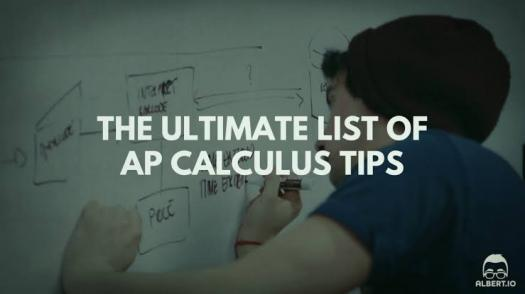10 Questions | Total Attempts: 139SettingsThe Advanced AP Calculus Test is an Advanced Placement calculus course consisting of a full high school academic year of work that is comparable to calculus courses in colleges and universities. The advanced test will prepare candidates and anyone that takes the test for the actual AP Calculus Test. Note: This is just a pre-test, it is not the actual test.

• 1.
Find X if In(x+5) = 3
• A.

E​​​​​​3​​​​​+5

• B.

E​​​​​​3​​​​​-5

• C.

3e​​​​​​3​​​​​+5

• D.

3e​​​​​​3​​​​​-5

• 2.
Find the volume of the solid formed by revolving the region bounded by y=2x-x2 and y=0 about the line x=1
• A.

16π/2

• B.

16π/3

• C.

16π/3

• D.

16π/5

• 3.
Evaluate tan{sin-1(2/3)}
• A.

2/3

• B.

2/√3

• C.

2/5

• D.

2/√5

• 4.
Find the area of the region bounded by the curves y=6-xand x+4
• A.

6/2

• B.

9/2

• C.

7/2

• D.

8/2

• 5.
Find an equation of the tangent line to y=-2x​​​​2+2x+1 at the point (-1,0)
• A.

Y=6x+6

• B.

Y=-3x-3

• C.

Y=-6x-6

• D.

Y=3x+3y=3x+3

• 6.
If f(x) = x​​​​​​2 and g(x) = x-3, find the composite function of f​​​​​​0​​​​​g
• A.

(x-3)2

• B.

X​​​​​​2+x-3

• C.

X​​​​​​2​​​​​-3

• D.

(x​​​​​​2​​​​​-3)2

• 7.
Find f'(0) of f(x)=sin​​​​2​​​(3-x)
• A.

2sin3cos3

• B.

-2sin3cos3

• C.

-2cos(3-x)

• D.

-2sin(3-x)

• 8.
If a ball is thrown into the air with a velocity of 40ft/s, its height in feet t seconds later is given by y=40t-16t2. Estimate the instantaneous velocity when t=2
• A.

22ft/s

• B.

28ft/s

• C.

20ft/s

• D.

24ft/s

• 9.
Find the absolute minimum of f(x) = 3x4-4x3 on (-2,3)
• A.

F​​​​​​min​​​=0

• B.

F​​​​​​min​​​=1

• C.

F​​​​​​min​​​=80

• D.

F​​​​​​min​​​=135

• 10.
Find the inverse of f(x)=x​​​​​​3​​​​​-1
• A.

1-3√x

• B.

1+3√x

• C.

3√x-1

• D.

3√x+1

• E.

None of the above

Related TopicsBack to top